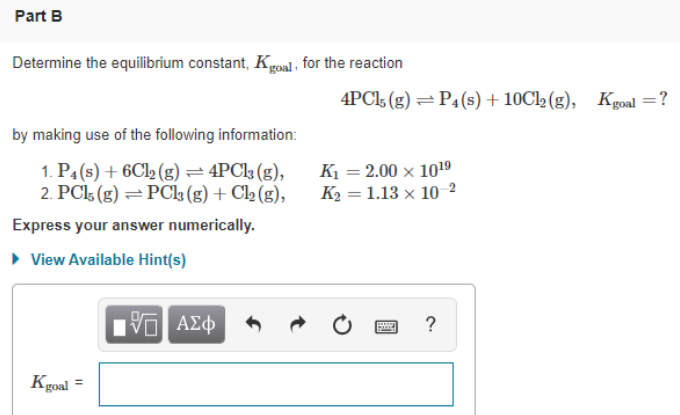# Determine the value of the equilibrium constant, Kgoal, for the reaction 4PCl5(g) ⇌ P4(s) + 10Cl2(g), Kgoal = ? by making use of the following information: 1. P4(s) + 6Cl2(g) ⇌ 4PCl3(g), K1 = 2.00 X 10^19 2. PCl5(g) ⇌ PCl3(g) + CL2(g), K2 = 1.13 X 10^-2 Express your answer numerically.